# Manually stacking photos

• LightningInAJar

#### LightningInAJar

Homework Statement
Not sure what this field is for.
Relevant Equations
Layer 1 (Bottom) = 100% opacity
Layer 2 = 50% opacity
Layer 3 = 33%
Layer 4 = 25%
Layer 5 = 20%
I was given the above opacity levels for stacking photos to create an equal average of all photos as a single photo. Can anyone explain how this works? I suspect if I had 4 photos labeled A to D I could combine A and B first, C and D second, then AB and CD last, all at 100% bottom layer and 50% top layer. Now that seems equal but tedious and limited. How does the math work in the given method above?

How do you think it might work

Is this really a homework problem without a statement of what they want you to do?

Homework statement is just please copy the text of the problem word for word, so we can be confident we are helping you solve the right problem.

Often the reason someone can't solve a problem is because when they try to say it in their own words they do it wrong.

I am not a student. This isn't actually homework. But whenever I post anywhere else in this forum they delete it as being too dumb for a serious research forum. Wasn't sure where else to go for what might be a simple math problem.

But whenever I post anywhere else in this forum they delete it as being too dumb for a serious research forum.
That's not true. By my count you have started 53 threads. Of these only two have been deleted. I didn't check all of your threads, but some of them could have been closed, which means they are still there, but members, including you, can't make new posts in them.

What people are objecting to in this thread is the ambiguity of what you have described. A clearer description of the question would likely lead to quicker answers.

Last edited:
@LightningInAJar, you STILL have not made any effort on your own. You should realize by now that this is NOT a "Q&A" forum where you just ask a question and get an answer. We expect to see some effort on your part. So, I ask again, how do you think it might work?

Its less of a math problem and more of a comprehension problem. The math aspect is trivial. The question is why those numbers?

You would use the same math to equally mix five vials of coloured liquid. If you did it stepwise.

Its less of a math problem and more of a comprehension problem. The math aspect is trivial. The question is why those numbers?

You would use the same math to equally mix five vials of coloured liquid. If you did it stepwise.
I don't understand that. If you wanted to mix 3 colors in equal parts wouldn't each be 1/3 parts each? But images in a particular order in software wouldn't be different? I tried to do the Harris Shutter effect in software using 100%, 50%, and 33% and although it looked interesting it seemed darker than original images.

Be systematic. You see 20% of layer 5. Can you calculate how much of layer 4 that you see?

1.0, Reduced to 0.5, Reduced to 0.16, Reduced to 0.04166

0.5, Reduced to 0.16, Reduced to 0.04166

0.33, Reduced to 0.04166

I tried to figure the influence of each layer added on top of every other layer minus the last one which is set to 25% which is clearly the only weight it has. But I don't know how it works. Seems to end on the same value, but the end value is like 1/24th. I can't visualize it.

Layer 5 is 20% opaque. That means that (1-20%)=4/5 of what is below is transmitted.

I don't understand that. If you wanted to mix 3 colors in equal parts wouldn't each be 1/3 parts each?
But you're assembling it step by step.

You mix the first two colours A and B 1:1 to get AB.

If you were to now mix AB and C at 1:1, then C would be over-represented.

You must mix AB and C at 2:1 to actually get equal representation from all colours.

Its not a perfect analogy to the layers. It might help it might not.

1.0, Reduced to 0.5, Reduced to 0.16, Reduced to 0.04166

0.5, Reduced to 0.16, Reduced to 0.04166

0.33, Reduced to 0.04166

I tried to figure the influence of each layer added on top of every other layer minus the last one which is set to 25% which is clearly the only weight it has. But I don't know how it works. Seems to end on the same value, but the end value is like 1/24th. I can't visualize it.
Start simple.
Say it's a pic of random red dots on a white field.
And say there's only 3 layers.

How opaque must the top layer be so that its contribution is no more than the botton two layers?

Since it's not really a homework problem, I think I can give an answer:
It looks like it works as follows:
You want the first and second layers to equally share the combined image, so give 50% to layer two, leaving 50% for layer 1.
You want layer 3 to share equally with the first two layers, so give it one-third, 33%, leaving the other 66% to be equally shared by layers 1 and 2.
You want layer 4 to share equally with the prior 3 layers, so give it one-fourth, 25%, leaving 75% to be shared equally by the prior 3 layers.

So the general formula is that layer n gets 1/n'th of the image or 1/n * 100%.
I am not saying that their logic will give the correct result, just that it seems to be their logic and it seems reasonable.

Yes, the algorithm is sound. It is also simple and universal.

Opacity of any layer n is simply n/100 expressed as a %. *

I suspect if I had 4 photos labeled A to D I could combine A and B first, C and D second, then AB and CD last, all at 100% bottom layer and 50% top layer. Now that seems equal but tedious and limited. How does the math work in the given method above?
That will only work with layer counts of 2n (i.e. 4, 8,16, etc.).
Try listing the steps for a stack of 7 layers. Or 31. Or 1023.

* Yes, I know FactChecker already explained that but I worked this out on the ceiling of my bedroom at 230AM last night, so I'm going to get my 15 seconds in thankyouverymuch!

Last edited:
Still can't visualize it as a table. Is there another way to draw it out? Maybe fractions or series of divisions. I mean when I do 3 images do I multiply the 50% and 100% by 1/3 or what? I actually had trouble even getting this opacity procedure from a photography forum because most of them use a Photoshop auto tool.

Layer 5 will get 1/5=20% of the image and leave 4/5 to be evenly split by the 4 lower layers.
Layer 4 will get 1/4=25% of the image amount allotted for that layer and below and will leave 3/4 to be evenly split by the 3 lower layers.
Layer 3 will get 1/3=33% of the image amount allotted for that layer and below and will leave 2/3 to be evenly split by the 2 lower layers.
Layer 2 will get 1/2=50% of the image amount allotted for that layer and below and will leave 1/2 for the lowest layer.
Layer 1 will get all=100% of the image amount allotted for that layer and below and will leave nothing for lower layers because there are no lower layers.

Still can't visualize it as a table. Is there another way to draw it out? Maybe fractions or series of divisions. I mean when I do 3 images do I multiply the 50% and 100% by 1/3 or what? I actually had trouble even getting this opacity procedure from a photography forum because most of them use a Photoshop auto tool.
Not sure what you're after. Theory or practice.

If practice, just use the formula:

Opacity of layer n is 100/n %

1 1/1 100
2 1/2 50
3 1/3 33
4 1/4 25
5 1/5 20

Layer 5 will get 1/5=20% of the image and leave 4/5 to be evenly split by the 4 lower layers.
Layer 4 will get 1/4=25% of the image amount allotted for that layer and below and will leave 3/4 to be evenly split by the 3 lower layers.
Layer 3 will get 1/3=33% of the image amount allotted for that layer and below and will leave 2/3 to be evenly split by the 2 lower layers.
Layer 2 will get 1/2=50% of the image amount allotted for that layer and below and will leave 1/2 for the lowest layer.
Layer 1 will get all=100% of the image amount allotted for that layer and below and will leave nothing for lower layers because there are no lower layers.
So layer 5 is 20% and subtract 5% of the 4 layers below it?

Not sure what you're after. Theory or practice.

If practice, just use the formula:

Opacity of layer n is 100/n %

1 1/1 100
2 1/2 50
3 1/3 33
4 1/4 25
5 1/5 20
I just want to see numerical proof that each image is represented equally.

I just want to see numerical proof that each image is represented equally.
I'm not sure a numerical proof would convince you.
Here's a graphical proof though.

Here are 4 theoretical images: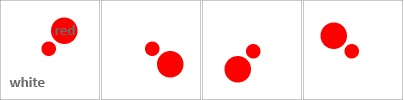Here is L1 at 100%:Here is
L1: 100%
+ L2: 50%.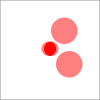Notice how both large dots are the same intensity (50%). Why?

Any given pixel, anywhere in the image, is getting
50% contribution from L1 and
50% contribution from L2.
That 'contribution' may be red or it may be white.

Examine this until you are satisfied why it is true.

Now, this is what happens with adding a third layer:
L1: 100%
+ L2: 50%

+ L3: 50%: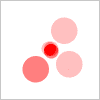Any given pixel is getting
50% from L3
but only
50% from L2 and L1 combined.

L3 is over-represented.

The only way to get an equal contribution from each layer is
L1: 100%
+ L2: 50%

+ L3: 33%: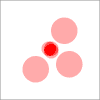All three large dots are equally intense.
Satisfy yourself why this must be true.

Finally, this is what happens with
L1: 100%
+ L2: 50%
+ L3: 33%

+ L4: 33%: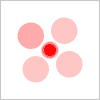Any given pixel is getting
33% from L4 +
67% from L3 and L2 and L1 combined.
That 67% is divided 3 ways - that means L1, L2 and L3 are only contributing ~22% each.
At 33%, L4 is over-represented.

The only way to get an equal contribution from each layer is
L1: 100%
+ L2: 50%
+ L3: 33%

+ L4: 25%: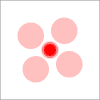#### Attachments

Last edited:
Here are 8 layers: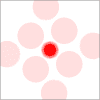L1: 100%
L2: 50%
L3: 33%
L4: 25%
L5: 20%
L6: 17%
L7: 14%
L8: 12%

Pick any pixel in the image.
Add up the contributions - one at a time from the bottom up, diluting the layers below - to that pixel's final colour.

(TIL: PF will only accept a max of 10 images per post.)

So layer 5 is 20% and subtract 5% of the 4 layers below it?
I wouldn't say it that way.
At layer 5, there are a total of 5 photos to merge into one photo, So each photo should get 1/5th = 20% of the image (opacity). Set the layer 5 opacity to 20% and leave the lower 4 unchanged. Calculate all the lower layer opacities the same way. (Layer 4 opacity = 1/4 = 25%, layer 3 opacity = 1/3 = 33%, layer 2 opacity = 1/2 = 50%, layer 1 opacity = 1/1 = 100%)

That is true for any number of layers.
If you are at layer 25, there are a total of 25 photos to merge into one photo. So set the layer 25 opacity at 1/25 = 4%. Calculate all the lower layers the same way. (Layer 24 opacity = 1/24 =~ 42%, layer 23 opacity = 1/23 =~ 43%, layer 22 opacity = 1/22 =~ 45%, ... , layer 5 opacity = 1/5 = 20%, ... )

I just want to see numerical proof that each image is represented equally.
I suggest that you prove the following using proof by induction

For each layer to be repesented equally, the nth layer should have an opacity 1/n. n=1,2,3,...

Start with n=2 where it is trivially true. Show it for n=k+1. Let us know how that works out for you.

•FactChecker
I just want to see numerical proof that each image is represented equally.
Simplify ##(1/5)L_5 + (4/5)( (1/4)L_4+(3/4)( (1/3)L_3+(2/3)( (1/2)L_2+(1/2)L_1) ) )##
A lot of the factors cancel out.
You get ##(1/5)L_5 + (1/5)L_4 + (1/5)L_3 + (1/5)L_2 + (1/5)L_1##, so all 5 layers are equally visible.
You can use @hutchphd 's post to prove that opacity of 100%/n for each layer ##L_n## works for any number of layers.

Last edited:
Here are 8 layers:
View attachment 312783
L1: 100%
L2: 50%
L3: 33%
L4: 25%
L5: 20%
L6: 17%
L7: 14%
L8: 12%

Pick any pixel in the image.
Add up the contributions - one at a time from the bottom up, diluting the layers below - to that pixel's final colour.

(TIL: PF will only accept a max of 10 images per post.)
I really appreciate the visuals. Not sure where you found those images. But I still don't know the math of why those fractions in particular are used. I know if there were 3 images and there was no order than each would be at 33%. It's like the bottom layer which is set to 100% if there were 5 layers total, then you need the influence of the top 4 layers to somehow add up to 80%. Not sure how to systematically know how to make that work.

I really appreciate the visuals. Not sure where you found those images.

But I still don't know the math of why those fractions in particular are used. I know if there were 3 images and there was no order than each would be at 33%.
You're going to need to go through it step by step before it will become intuitive.

Review post 22, from the top, down to where it says: "L3 is over-represented."
Convince yourself why the only way to get this: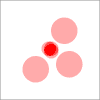is 100% / 50% / 33%.

If it doesn't makes sense, tell us exactly where it goes off the rails.

#### Attachments

I really appreciate the visuals. Not sure where you found those images. But I still don't know the math of why those fractions in particular are used. I know if there were 3 images and there was no order than each would be at 33%. It's like the bottom layer which is set to 100% if there were 5 layers total, then you need the influence of the top 4 layers to somehow add up to 80%. Not sure how to systematically know how to make that work.
Do the following. Simplify things to only 3 layers. Assume each layers emits 1*opacity amount of light (so layer 1 emits 1 unit, layer 2 emits 1/2 units, layer 3 emits 1/3 units, etc). Then write down how much light from layer 1 makes it through layer 2. Then write how much of the remaining light from layer 1 makes it through layer 3.
Then write down how much light from layer 2 makes it through layer 3. Then write down how much light there is from layer 3.

Ok, finally figured it out. Top to bottom. I basically take the value of the percentage and divide by 100 to get 1 or less decimal and subtract from 1 to get opposite portion and multiply that by everything below it. At the end everything was 20% left.

20%
25%, 20%
33.3%, 26.66%, 20%
50%, 40%, 30%, 20%
100%, 80%, 60%, 40%, 20%

Ok, finally figured it out. Top to bottom. I basically take the value of the percentage and divide by 100 to get 1 or less decimal and subtract from 1 to get opposite portion and multiply that by everything below it. At the end everything was 20% left.

20%
25%, 20%
33.3%, 26.66%, 20%
50%, 40%, 30%, 20%
100%, 80%, 60%, 40%, 20%
I agree although I could quibble about some details of how you stated it.
It might seem like magic that they all end up with 20% ( = 1/5).
IMO, it is easier to accept if you look at post #26 and notice how many factors cancel out.
Start with the whole thing, as you described it, with none of the multiplications or divisions calculated out:
##(1/5)L_5 + (4/5)( (1/4)L_4+(3/4)( (1/3)L_3+(2/3)( (1/2)L_2+(1/2)L_1) ) )##

Look at the last term above and cancel the multiply/divide by 2 to get this:
##(1/5)L_5 + (4/5)( (1/4)L_4+(3/4)( (1/3)L_3+(1/3)( L_2+L_1) ) )##
and combine the last two terms:
##(1/5)L_5 + (4/5)( (1/4)L_4+(3/4)( (1/3)(L_3+L_2+L_1) ) )##

Look at the last term above and cancel the multiply/divide by 3 to get this:
##(1/5)L_5 + (4/5)( (1/4)L_4+(1/4)( L_3+L_2+L_1 ) )##
and combine the last two terms:
##(1/5)L_5 + (4/5)( (1/4)(L_4+L_3+L_2+L_1 ) )##

Look at the last term above and cancel the multiply/divide by 4 to get this:
##(1/5)L_5 + (1/5)( L_4+L_3+L_2+L_1 )##
and combine the last two terms:
##(1/5)(L_5+L_4+L_3+L_2+L_1 )##

So every multiplier/divisor canceled out except the divisor for the highest level.

Last edited:
Ok, finally figured it out. Top to bottom. I basically take the value of the percentage and divide by 100 to get 1 or less decimal and subtract from 1 to get opposite portion and multiply that by everything below it. At the end everything was 20% left.

20%
25%, 20%
33.3%, 26.66%, 20%
50%, 40%, 30%, 20%
100%, 80%, 60%, 40%, 20%
What do these numbers represent?

For example, what exactly is line 3? Is that meant to be the opacities for 3 layers? It isn't.

While we're at it, what is line 1? Or 2?

The opacities should not be linear. You should get 100 50 33 25 20 17.

Last edited:
Ok, finally figured it out. Top to bottom. I basically take the value of the percentage and divide by 100 to get 1 or less decimal and subtract from 1 to get opposite portion and multiply that by everything below it. At the end everything was 20% left.

20%
25%, 20%
33.3%, 26.66%, 20%
50%, 40%, 30%, 20%
100%, 80%, 60%, 40%, 20%
As Dave said, I don't know what these percentages are supposed to represent.
A single layer should be 100% opacity, not 20%.

The entire point of the opacities is that the 'light' from underlying layers gets absorbed partially by the layers above.

For a two layer stack: layer 1 loses 50% of its light as it passes through layer 2, because layer 2 has 50% opacity, which means it let's 50% of the underlying light through, and emits some quantity that's equal to that 50%. If we assume that layer 1 emits 100 units of light then 50 units will be absorbed by layer 2, the other 50 units will make it through, and layer 2 will emit another 50 units of light. The total light is then 100 units, with equal contribution from both layers. This makes the final image an average of the original two.

For three layers you have:
Layer 1 emits 100 units of light.
100*1/2 = 50 units after passing through layer two.
Since the opacity of layer 3 is 1/3 (the same as 2/3 transparency), this means that 2/3 of the light makes it through.
50*2/3 = 33 1/3 units from layer 1 makes it through 3.

Layer 2 emits 50 units of light.
50*2/3 = 33 1/3 units of light from layer 2 that makes it through layer 3.

Layer 3 emits 33 1/3 units of light. All of it makes it to the final image.

Each layer thus has equal amounts of 'light' make it through to form the final stacked image. This is again an average since each image has an equal contribution.

What do these numbers represent?

For example, what exactly is line 3? Is that meant to be the opacities for 3 layers? It isn't.

While we're at it, what is line 1? Or 2?

The opacities should not be linear. You should get 100 50 33 25 20 17.
20%
25%, 20%
33.3%, 26.66%, 20%
50%, 40%, 30%, 20%
100%, 80%, 60%, 40%, 20%

100% is on bottom and 20% on top. Since 20% is the top opacity it leaves 80% of all of the layers below it. So .8 times 100% brings it down to 80%, 50% down to 40%, etc. And then I apply layer 2 to 3, 4, and 5 by multiplying by .75 and continue on until everything is brought down to 20% each.

20%
25%, 20%
33.3%, 26.66%, 20%
50%, 40%, 30%, 20%
100%, 80%, 60%, 40%, 20%

100% is on bottom and 20% on top. Since 20% is the top opacity it leaves 80% of all of the layers below it. So .8 times 100% brings it down to 80%, 50% down to 40%, etc. And then I apply layer 2 to 3, 4, and 5 by multiplying by .75 and continue on until everything is brought down to 20% each.
But why?? Its mystifying what you are trying to do.

What is it about the last 2 dozen posts of tutelage that makes you not want to do it this way?

•hutchphd